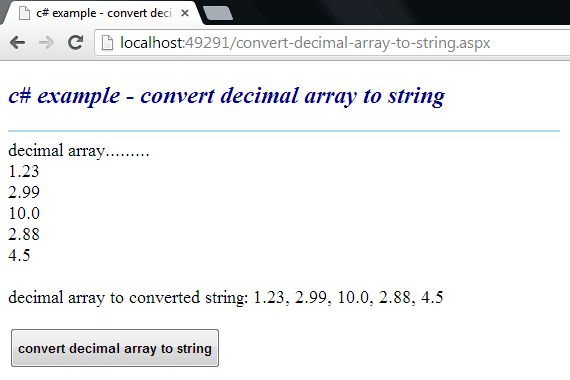### c# - How to convert a decimal array to a string

Convert a Decimal Array to a String
The Array class provides methods for creating, manipulating, searching, and sorting arrays. The Array class is not part of the System.Collections namespaces. However, it is still considered a collection because it is based on the IList interface. An element is a value in an Array. The length of an Array is the total number of elements it can contain. The Array has a fixed capacity.

The following .net c# tutorial code demonstrates how we can convert a Decimal Array instance to a String object. That means we will get a String object from Decimal Array elements. In this .net code, we will join the Decimal Array elements with a specified separator and build an instance of the String object. Here we will use the String class Join() method.

The String class Join() method concatenates the elements of a specified array or the members of a collection, using the specified separator between each element or member.

The String class Join(String, String[]) method overload concatenates all the elements of a String array, using the specified separator between each element. The Join (string? separator, params string?[] value) method has two parameters those are separator and value. Here the separator parameter is the String to use as a separator. And the value parameter is an Array that contains the elements to concatenate.

So, using this method overload .net developers can join Decimal Array elements to a String instance. Here we will pass “, ” for the separator parameter value and the Decimal Array for the value parameter. The String(separator, value) method returns a String that consists of the elements in value delimited by the separator String or an empty String if our provided Decimal Array contains zero elements.

The String Join(separator, value) method throws ArgumentNullException when the provided value is null. This method also throws OutOfMemoryException if the length of the resulting string overflows the maximum allowed length (Int32.MaxValue).

So finally, using this String class Join(String, String[]) method the .net c# developers can convert a Decimal Array to a String.
convert-decimal-array-to-string.aspx
``````
<%@ Page Language="C#" AutoEventWireup="true"%>

<!DOCTYPE html>
<script runat="server">
protected void Button1_Click(object sender, System.EventArgs e)
{
decimal[] decimalarray = new decimal[]
{
1.23M,
2.99m,
10.0M,
2.88M,
4.5M
};

Label1.Text = "decimal array.........<br />";
foreach (decimal d in decimalarray)
{
Label1.Text += d.ToString() + "<br />";
}

string convertedstring = String.Join(", ", decimalarray);
Label1.Text += "<br />decimal array to converted string: " + convertedstring;
}
</script>

<html xmlns="http://www.w3.org/1999/xhtml">
<title>c# example - convert decimal array to string</title>
<body>
<form id="form1" runat="server">
<div>
<h2 style="color:DarkBlue; font-style:italic;">
c# example - convert decimal array to string
</h2>
<hr width="550" align="left" color="LightBlue" />

<asp:Label
ID="Label1"
runat="server"
Font-Size="Large"
>
</asp:Label>
<br /><br />
<asp:Button
ID="Button1"
runat="server"
Text="convert decimal array to string"
OnClick="Button1_Click"
Height="40"
Font-Bold="true"
/>
</div>
</form>
</body>
</html>
``````More c# examples